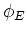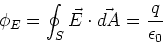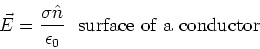PHY294H - Lecture 6

Gauss' Law states that the electric flux,, through a closed surface, S, is equal to the amount of charged enclosed by (i.e. inside) the surface S.(1)

Some questions and subtleties:

- Why don't charges that are outside the surface, S, make a contribution to?

- If the total charge inside a closed surface (S) is zero, is the electric field everywhere on the surface zero?

A unique feature of a charged piece of metal is that, no matter what its shape is and if current is zero, then the electric field inside the piece of metal has to be zero. Free charges in the metal go to the surfaces of the metal and arrange themselves so that the electric field is zero everywhere inside the metal. Electrostatics is a linear theory, so the equilibrium charge distribution is unique, ie. there is only one arrangement of the charges on the surface which produces zero electric field everywhere inside the metal.

If there is a component of the electric field at the surface of a conductor which is parallel to the surface, then charge would flow. At equilibrium there is no current in electrostatics, so the electric field at the surface of a conductor is perpendicular to the surface of the metal, everywhere. ie. the electric field is in the direction of the surface normal. It is then possible to draw a small Gaussian surface around a surface element and to show that,(2)

Closed metal surface - Faraday cage

An interesting effect is that a closed metal surface, no matter what it's shape, screens out external sources of electric field. Many high tech experiments are done inside a metal room, called a Faraday cage, so that effects due to stray electric fields are avoided. Stated another way, if there are no charges inside a closed metal surface, then the electric field inside the metal surface is zero everywhere. This is a much more general result than the shell theorems which apply only to uniform spherical shells of charge. Proving this result is beyond the level of this course, so I have to use the dreaded phrase it can be shown''.

Demo: Faraday's ice pail - a test of Gauss' law